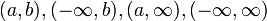# Differentiable functions form a vector space

## Statement for single differentiability

### Differentiability on an open interval version

Consider an interval$I$ that is open but possibly infinite in one or both directions (i.e., an interval of the form$(a,b),(-\infty,b),(a,\infty),(-\infty,\infty)$). A differentiable function on$I$ is a function whose derivative exists at every point of$I$.

The differentiable functions on$I$ form a real vector space, in the following sense:

• Additive closure: If$f$ and$g$ are differentiable functions on$I$, the pointwise sum of functions$f + g$ is also differentiable on$I$.
• Scalar multiples: If$f$ is a differentiable function on$I$ and$\lambda \in \R$, then$\lambda f$ is a differentiable function on$I$.

## Statement for multiple differentiability

### Differentiable a fixed finite number of times

All the above versions hold if we replace differentiable functions by multiply differentiable functions, i.e.,$k$ times differentiable functions, where$k$ is a fixed positive integer (this means that the$k^{th}$ derivative -- see higher derivative -- exists). The interval version reads as follows:

For any interval$I$ and positive integer$k$, the$k$ times differentiable functions on$I$ form a real vector space, in the following sense:

• Additive closure: If$f$ and$g$ are$k$ times differentiable functions on$I$, the pointwise sum of functions$f + g$ is also$k$ times differentiable on$I$.
• Scalar multiples: If$f$ is a$k$ times differentiable function on$I$ and$\lambda \in \R$, then$\lambda f$ is a$k$ times differentiable function on$I$.

### Infinitely differentiable

The above also holds if we replace differentiable by the notion of infinitely differentiable. An infinitely differentiable function is a function that is$k$ times differentiable for all$k$. The interval version reads as follows:

For any interval$I$, the infinitely differentiable functions on$I$ form a real vector space, in the following sense:

• Additive closure: If$f$ and$g$ are infinitely differentiable functions on$I$, the pointwise sum of functions$f + g$ is also infinitely differentiable on$I$.
• Scalar multiples: If$f$ is infinitely differentiable function on$I$ and$\lambda \in \R$, then$\lambda f$ is infinitely differentiable function on$I$.